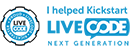## Floating point rounding error & how to overcome?

Using LiveCode in research or teaching of post secondary topics? Share your interests with other academics.

Moderators: Klaus, FourthWorld, heatherlaine, kevinmiller, robinmiller

rumplestiltskin
Posts: 222
Joined: Wed Jun 21, 2006 7:33 pm
Location: West of the Pecos
Contact:

### Floating point rounding error & how to overcome?

This is probably somewhat rare but I've noticed that using a number with 7 decimal places in any sort of calculation yields a number (prior to the calculation) that is rounded. For example:

answer round("29.9999999",7) * 1 yields an answer of 30 -- (seven decimal places)
-whereas-
answer round("29.999999",7) * 1 yields an answer of 29.999999 -- (six decimal places)

(I can increase the precision argument from 7 to 15 and it makes no difference.)

Note that using the trunc() function yields an answer of 30 for the first example but 29 for the second.

I've been using the offset function to determine if there is a decimal point present in the string provided by the user and, if so, then truncate the -string- down to the integer portion; then I may use the integer portion that remains in whatever calculations are necessary.

Is there an easier way to, perhaps, simply set the precision high enough where I can simply use the trunc() function for all numbers a user could possible enter (within reason)?

Thanks,
Barry

Dixie
Livecode Opensource BackerPosts: 1336
Joined: Sun Jul 12, 2009 10:53 am
Location: Bordeaux, France

### Re: Floating point rounding error & how to overcome?

Barry,

From 'numberformat' in the dictionary...

Since LiveCode does not use decimal numbers for its internal calculations (for reasons of speed), the decimal representation of a number is sometimes slightly off the correct number. For example, 10^-1 is equal to 0.1, but is calculated (to eighteen decimal places) as 0.100000000000000006. Because of this, setting the numberFormat to specify many decimal places after the decimal point may produce unexpected results in a statement that tests for an exact number. To prevent this, either avoid setting the numberFormat to a value more precise than you need, or use the abs function instead of the = operator to test equality...

be well,

Dixie

rumplestiltskin
Posts: 222
Joined: Wed Jun 21, 2006 7:33 pm
Location: West of the Pecos
Contact:

### Re: Floating point rounding error & how to overcome?

Turns out if you set the numberformat property to, let's say, 14 decimal places, calculations work as expected -if- you keep any numbers the program sees to 14 or fewer decimal places. The moment you give the program a number with 15 decimal places (or just one more than the numberformat - 7 in the case of the default settings), calculations don't work out properly.

I was running a simple game with some students and the stack would ask them their age. One of the students entered 29.9999999 and the handler took the trunc of the provided number which, of course, would end up being rounded (prior to the actual calculation but, presumably, as soon as LiveCode was directed to handle the user's entry as a number) to 30 instead of trunc'd to 29. If the number had only 6 decimal places (29.999999), the calculation worked properly.

I think the dictionary could provide a more transparent explanation if it left out technobabble. Maybe a link to the more verbose explanation could be provided for those with an interest.In the grand scheme of things, this is a minute problem.